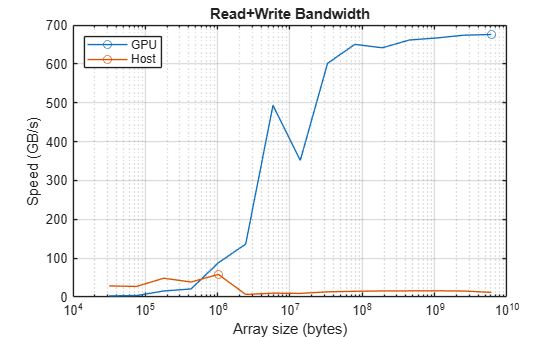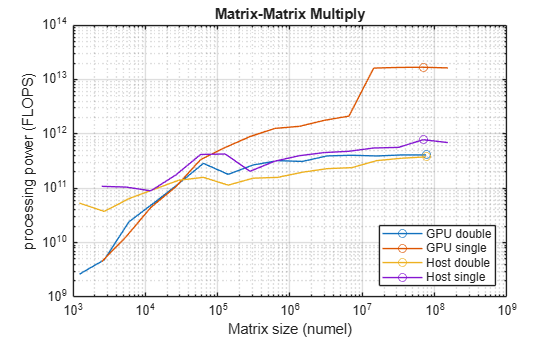Measuring GPU Performance

This example shows how to measure some of the key performance characteristics of a GPU.

GPUs can be used to speed up certain types of computations. However, GPU performance varies widely between different GPU devices. In order to quantify the performance of a GPU, three tests are used:

• How quickly can data be sent to the GPU or read back from it?

• How fast can the GPU kernel read and write data?

• How fast can the GPU perform computations?

After measuring these, the performance of the GPU can be compared to the host CPU. This provides a guide as to how much data or computation is required for the GPU to provide an advantage over the CPU.

Setup

gpu = gpuDevice();
fprintf('Using a %s GPU.\n', gpu.Name)
sizeOfDouble = 8; % Each double-precision number needs 8 bytes of storage
sizes = power(2, 14:28);
Using a Tesla K40c GPU.

Testing host/GPU bandwidth

The first test estimates how quickly data can be sent to and read from the GPU. Because the GPU is plugged into the PCI bus, this largely depends on how fast the PCI bus is and how many other things are using it. However, there are also some overheads that are included in the measurements, particularly the function call overhead and the array allocation time. Since these are present in any "real world" use of the GPU, it is reasonable to include these.

In the following tests, memory is allocated and data is sent to the GPU using the gpuArray function. Memory is allocated and data is transferred back to host memory using gather.

Note that PCI express v3, as used in this test, has a theoretical bandwidth of 0.99GB/s per lane. For the 16-lane slots (PCIe3 x16) used by NVIDIA's compute cards this gives a theoretical 15.75GB/s.

sendTimes = inf(size(sizes));
gatherTimes = inf(size(sizes));
for ii=1:numel(sizes)
numElements = sizes(ii)/sizeOfDouble;
hostData = randi([0 9], numElements, 1);
gpuData = randi([0 9], numElements, 1, 'gpuArray');
% Time sending to GPU
sendFcn = @() gpuArray(hostData);
sendTimes(ii) = gputimeit(sendFcn);
% Time gathering back from GPU
gatherFcn = @() gather(gpuData);
gatherTimes(ii) = gputimeit(gatherFcn);
end
sendBandwidth = (sizes./sendTimes)/1e9;
[maxSendBandwidth,maxSendIdx] = max(sendBandwidth);
fprintf('Achieved peak send speed of %g GB/s\n',maxSendBandwidth)
gatherBandwidth = (sizes./gatherTimes)/1e9;
[maxGatherBandwidth,maxGatherIdx] = max(gatherBandwidth);
fprintf('Achieved peak gather speed of %g GB/s\n',max(gatherBandwidth))
Achieved peak send speed of 6.18519 GB/s
Achieved peak gather speed of 3.31891 GB/s

On the plot below, the peak for each case is circled. With small data set sizes, overheads dominate. With larger amounts of data the PCI bus is the limiting factor.

hold off
semilogx(sizes, sendBandwidth, 'b.-', sizes, gatherBandwidth, 'r.-')
hold on
semilogx(sizes(maxSendIdx), maxSendBandwidth, 'bo-', 'MarkerSize', 10);
semilogx(sizes(maxGatherIdx), maxGatherBandwidth, 'ro-', 'MarkerSize', 10);
grid on
title('Data Transfer Bandwidth')
xlabel('Array size (bytes)')
ylabel('Transfer speed (GB/s)')
legend('Send to GPU', 'Gather from GPU', 'Location', 'NorthWest')Testing memory intensive operations

Many operations do very little computation with each element of an array and are therefore dominated by the time taken to fetch the data from memory or to write it back. Functions such as ones, zeros, nan, true only write their output, whereas functions like transpose, tril both read and write but do no computation. Even simple operators like plus, minus, mtimes do so little computation per element that they are bound only by the memory access speed.

The function plus performs one memory read and one memory write for each floating point operation. It should therefore be limited by memory access speed and provides a good indicator of the speed of a read+write operation.

memoryTimesGPU = inf(size(sizes));
for ii=1:numel(sizes)
numElements = sizes(ii)/sizeOfDouble;
gpuData = randi([0 9], numElements, 1, 'gpuArray');
plusFcn = @() plus(gpuData, 1.0);
memoryTimesGPU(ii) = gputimeit(plusFcn);
end
memoryBandwidthGPU = 2*(sizes./memoryTimesGPU)/1e9;
[maxBWGPU, maxBWIdxGPU] = max(memoryBandwidthGPU);
fprintf('Achieved peak read+write speed on the GPU: %g GB/s\n',maxBWGPU)
Achieved peak read+write speed on the GPU: 186.494 GB/s

Now compare it with the same code running on the CPU.

memoryTimesHost = inf(size(sizes));
for ii=1:numel(sizes)
numElements = sizes(ii)/sizeOfDouble;
hostData = randi([0 9], numElements, 1);
plusFcn = @() plus(hostData, 1.0);
memoryTimesHost(ii) = timeit(plusFcn);
end
memoryBandwidthHost = 2*(sizes./memoryTimesHost)/1e9;
[maxBWHost, maxBWIdxHost] = max(memoryBandwidthHost);
fprintf('Achieved peak read+write speed on the host: %g GB/s\n',maxBWHost)

% Plot CPU and GPU results.
hold off
semilogx(sizes, memoryBandwidthGPU, 'b.-', ...
sizes, memoryBandwidthHost, 'r.-')
hold on
semilogx(sizes(maxBWIdxGPU), maxBWGPU, 'bo-', 'MarkerSize', 10);
semilogx(sizes(maxBWIdxHost), maxBWHost, 'ro-', 'MarkerSize', 10);
grid on
xlabel('Array size (bytes)')
ylabel('Speed (GB/s)')
legend('GPU', 'Host', 'Location', 'NorthWest')
Achieved peak read+write speed on the host: 40.2573 GB/sComparing this plot with the data-transfer plot above, it is clear that GPUs can typically read from and write to their memory much faster than they can get data from the host. It is therefore important to minimize the number of host-GPU or GPU-host memory transfers. Ideally, programs should transfer the data to the GPU, then do as much with it as possible while on the GPU, and bring it back to the host only when complete. Even better would be to create the data on the GPU to start with.

Testing computationally intensive operations

For operations where the number of floating-point computations performed per element read from or written to memory is high, the memory speed is much less important. In this case the number and speed of the floating-point units is the limiting factor. These operations are said to have high "computational density".

A good test of computational performance is a matrix-matrix multiply. For multiplying twomatrices, the total number of floating-point calculations is.

Two input matrices are read and one resulting matrix is written, for a total ofelements read or written. This gives a computational density of (2N - 1)/3 FLOP/element. Contrast this with plus as used above, which has a computational density of 1/2 FLOP/element.

sizes = power(2, 12:2:24);
N = sqrt(sizes);
mmTimesHost = inf(size(sizes));
mmTimesGPU = inf(size(sizes));
for ii=1:numel(sizes)
% First do it on the host
A = rand( N(ii), N(ii) );
B = rand( N(ii), N(ii) );
mmTimesHost(ii) = timeit(@() A*B);
% Now on the GPU
A = gpuArray(A);
B = gpuArray(B);
mmTimesGPU(ii) = gputimeit(@() A*B);
end
mmGFlopsHost = (2*N.^3 - N.^2)./mmTimesHost/1e9;
[maxGFlopsHost,maxGFlopsHostIdx] = max(mmGFlopsHost);
mmGFlopsGPU = (2*N.^3 - N.^2)./mmTimesGPU/1e9;
[maxGFlopsGPU,maxGFlopsGPUIdx] = max(mmGFlopsGPU);
fprintf(['Achieved peak calculation rates of ', ...
'%1.1f GFLOPS (host), %1.1f GFLOPS (GPU)\n'], ...
maxGFlopsHost, maxGFlopsGPU)
Achieved peak calculation rates of 72.5 GFLOPS (host), 1153.3 GFLOPS (GPU)

Now plot it to see where the peak was achieved.

hold off
semilogx(sizes, mmGFlopsGPU, 'b.-', sizes, mmGFlopsHost, 'r.-')
hold on
semilogx(sizes(maxGFlopsGPUIdx), maxGFlopsGPU, 'bo-', 'MarkerSize', 10);
semilogx(sizes(maxGFlopsHostIdx), maxGFlopsHost, 'ro-', 'MarkerSize', 10);
grid on
title('Double precision matrix-matrix multiply')
xlabel('Matrix size (numel)')
ylabel('Calculation Rate (GFLOPS)')
legend('GPU', 'Host', 'Location', 'NorthWest')Conclusions

These tests reveal some important characteristics of GPU performance:

• Transfers from host memory to GPU memory and back are relatively slow.

• A good GPU can read/write its memory much faster than the host CPU can read/write its memory.

• Given large enough data, GPUs can perform calculations much faster than the host CPU.

It is notable that in each test quite large arrays were required to fully saturate the GPU, whether limited by memory or by computation. GPUs provide the greatest advantage when working with millions of elements at once.

More detailed GPU benchmarks, including comparisons between different GPUs, are available in GPUBench on the MATLAB® Central File Exchange.

Parallel Computing Toolbox DocumentationGet trial now